• +91 9971497814
• info@interviewmaterial.com

# RD Chapter 20- Surface Areas and Volume of A Right Circular Cone Ex-20.1 Interview Questions Answers

### Related Subjects

Question 1 : Find the curved surface area of a cone, if its slant height is 60 cm and the radius of its base is 21 cm.

Radius of the base of the cone = 21 cm
and slant height (l) = 60 cm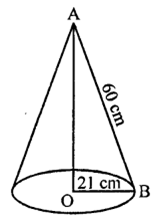Question 2 : The radius of a cone is 5 cm and vertical height is 12 cm. Find the area of the curved surface.

Radius of the base of a cone = 5 cm
Vertical height (h) = 12 cm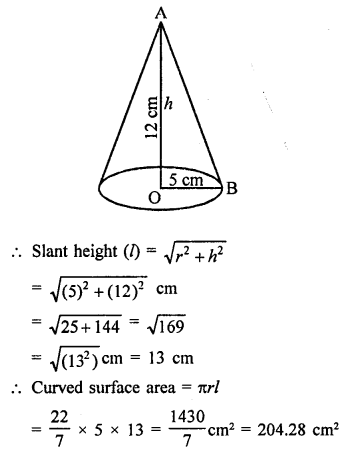Question 3 : The radius of a cone is 7 cm and area of curved surface is 176 cm2. Find the slant height.

Curved surface area of a cone = 176 cm2
and radius (r) = 1 cm2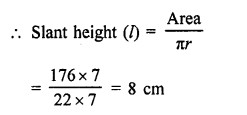Question 4 : The height of a cone is 21 cm. Find the area of the base if the slant height is 28 cm.

Heightof the cone (h) = 21 cm
Slant height (l) = 28 cm
l2 = r2 +h2
r2 = l2–h2 = (28)2 –(21 )2
784 –441 = 343 …(i)
Now area of base = πr2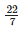x343 [From (i)]

= 22 x 49 = 1078 cm2

Question 5 : Find the total surface area of a right circular cone with radius 6 cm and height 8 cm.

Radius of base of cone (r) = 6 cm
and height (h) = 8 cm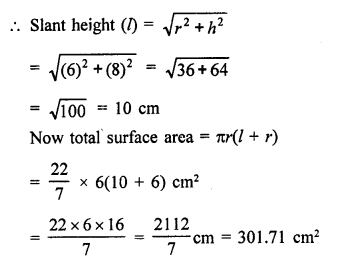Question 6 : Find the curved surface area of a cone with base radius 5.25 cm and slant height 10 cm. [NCERT]

Radius of base of a cone (r) = 5.25 cm
and slant height (l) = 10 cm
Curved surface area = πrl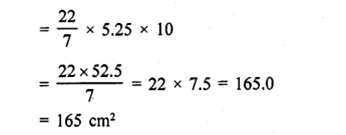Question 7 : Find the total surface area of a cone, if its slant height is 21 m and diameter of its base is 24 m.

Slant height of a cone (l) = 21 m
and diameter of its base = 24 m
∴ Radius (r) = 24/2 = 12 m
Now total surface area = πr(l + r)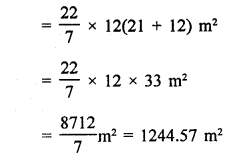Question 8 : The area of the curved surface of a cone is 60π cm2. If the slant height of the cone be 8 cm, find the radius of the base.

Curved surface area of a cone = 6071 cm2
Slant height (l) = 8 cm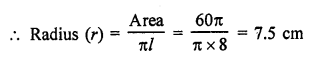Question 9 : The curved surface area of a cone is 4070 cm2 and its diameter is 70 cm. What is the slant height ? (Use π = 22/7).

Surface area of a cone = 4070 cm2
Diameter of its base = 70 cm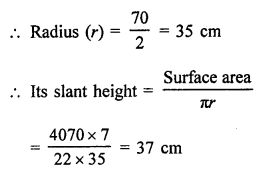Question 10 : The radius and slant height of a cone are in the ratio of 4 : 7. If its curved surface area is 792 cm2, find its radius. (Use π = 22/7)

Answer 10 : Curved surface area of a cone = 792 cm2
Ratio in radius and slant height = 4:7
Then slant height = 7x
Curved surface area πrl =792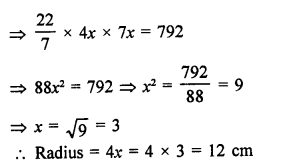Todays Deals### RD Chapter 20- Surface Areas and Volume of A Right Circular Cone Ex-20.1 Contributorskrishan

Name:
Email:

# Latest News# 9000 interview questions in different categories